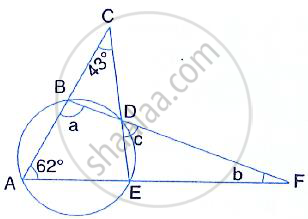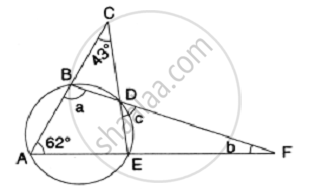Share

# In the Given Figure, ∠Ace = 43° and ∠Caf = 62° ; Find the Values of A, B and C. - Mathematics

Course

#### Question

In the given figure, ∠ACE = 43° and ∠CAF = 62° ; Find the values of a, b and c.#### SolutionNow, ∠ACE = 43° and ∠CAF = 62°     [given]
In ΔAEC
∴ ∠ACE + ∠CAE + ∠AEC = 180°
⇒ 43° + 62° + ∠AEC = 180°
⇒ 105° + ∠AEC = 180°
⇒ ∠AEC = 180° -105° = 75°
Now, ∠ABD + ∠AED = 180°
[Opposite angles of a cyclic quad and ∠AED = ∠AEC]
⇒ a + 75° = 180°
⇒ a = 180° - 75°
⇒ a = 105°
∠EDF = ∠BAF

∴   c = 62°       [Angles in the alternate segments]

In ΔBAF,a + 62° + b = 180°
⇒ 105° + 62° + b = 180°
⇒ 167° + b = 180°
⇒ b = 180° -167° = 13°
Hence, a = 105°, b = 13° and c = 62°

Is there an error in this question or solution?